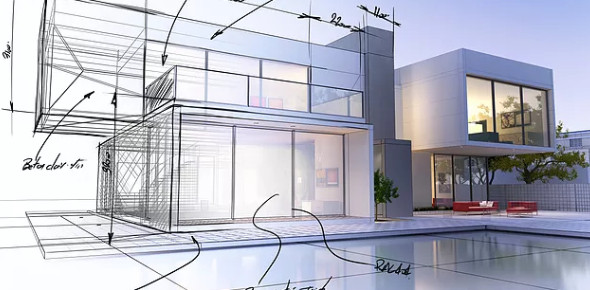# Structural Engineering Exam Quiz!

40 Questions | Total Attempts: 11Settings.

Questions and Answers
• 1.
An alternative way of providing a 90-degree hook bend on a 36mm bar without the use of a machine bender?
• A.

Heating the bar for easy bending

• B.

Cutting a piece of bar and welding it 90 degree on vertical bar

• C.

Bending two smaller diameter bars which are equivalent to 36mm bar manually

• 2.
A type of half-lapped joint that resists tension?
• A.

Lock splice

• B.

Scarf splice

• C.

Square splice

• 3.
Cutting of concrete pouring on a beam should be?
• A.

Perpendicular to the span

• B.

Parallel to the span

• C.

Diagonally across the span

• 4.
Refer to the portion of a beam where bending moment changes from positive to negative?
• A.

Inflection point

• B.

Mid point

• C.

Top beam

• 5.
The support used in steel decking?
• A.

Scaffolding

• B.

Bracing system

• C.

Props

• 6.
A bar used to hold the reinforcement on a beam?
• A.

Tie bar

• B.

Web

• C.

Lateral ties

• 7.
If the riser is 18cm, find the tread width using riser / tread proportion formula (R) (T) = 75?
• A.

26.88 cm

• B.

28.66 cm

• C.

32.68 cm

• 8.
Specification of wide flange as W-12x24 means?
• A.

Width and height

• B.

Weight and height

• C.

Thickness x width x height

• 9.
This is used for extending 32mm reinforcing bars?
• A.

Coupler

• B.

Splicing

• C.

Plate

• 10.
Using 1/2" thk. Plywood and 2' x 3' formworks, what is the economical section of a column?
• A.

500mmx500 mm

• B.

600x600 mm

• C.

700x700 mm

• 11.
Inclined structural member that supports the steps of a stair?
• A.

Flight

• B.

Curtain step

• C.

Carriage

• 12.
Rigid connection or anchorage at support of a beam?
• A.

Restraint

• B.

Pre-stressed

• C.

Tied beam

• 13.
What is the thickness of a two way slab with a dimension of 5000x6000 mm?
• A.

100mm

• B.

122mm

• C.

150mm

• 14.
Width of prestressed beam with a span of 8.0 m on light loadings?
• A.

250mm

• B.

500mm

• C.

400mm

• 15.
Street line elevation is +10.00m, the reference point is 2.00m above street line, the natural grade line is 3.00 below the reference point. Column footing depth is 2.00m from the natural grade line. What is the elevation of the foundation bed?
• A.

+9.00m

• B.

+5.00m

• C.

+7.00m

• 16.
If the water content of the soil is high, what is to ideal way to do with the footing?
• A.

Deeper

• B.

Wider

• C.

Thicker

• 17.
In precast construction, bearing pad used for joint that rely on gravity by placing a beam on a corbel of a column?
• A.

Synthetic rubber

• B.

High density plastic

• C.

padding foam

• 18.
Jack rafter between the hip and the valley rafters?
• A.

Hip jack rafter

• B.

Cripple jack rafter

• C.

Valley jack rafter

• 19.
Providing bolts on two girders within the span should have?
• A.

Concrete spacer

• B.

Slipper

• C.

Wood block

• 20.
Stone placed on a slope to prevent erosion?
• A.

Rip rap

• B.

Flag stone

• C.

Border stone

• 21.
Used to hold horizontal batten on top of corrugated sheathing?
• A.

Corrugated G.I. sheet

• B.

Batten nail

• C.

Vertical batten

• 22.
Distributes the load of the column equally among the piles?
• A.

Footing

• B.

Pile cap

• C.

Pile foundation

• 23.
Reinforcing bars provided within the span of two columns on combined footing?
• A.

Top bar

• B.

Web bars

• C.

Temperature bars

• 24.
A joint between adjacent parts of a structure which permits movement between them?
• A.

Control joint

• B.

Construction joint

• C.

Contraction joint

• 25.
The distance between inflection point in the column when it breaks?
• A.

Developed length

• B.

Effective length

• C.

Equivalent length

Related Topics